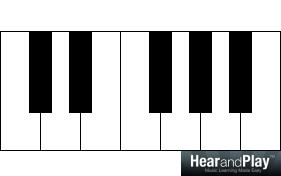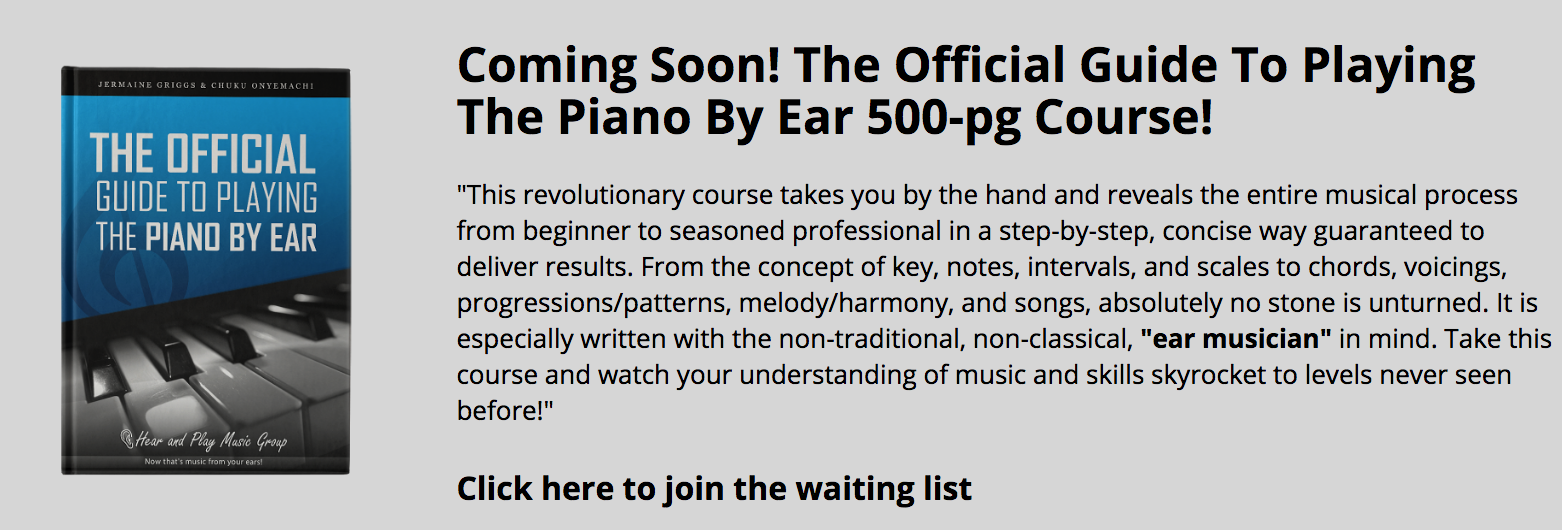• # Who Else Wants To Learn How To Invert Intervals?If you’re interested in learning how to invert intervals, then this lesson is for you.

In the next ten minutes or so, you’ll be learning how intervals can be inverted and most importantly, how the quality and quantity of an interval changes when inverted.

At the end of this lesson, with your knowledge of the inversion of intervals, you’ll understand the relationship between different qualities and quantities of intervals.

Before jumping into all that, let’s do a review on intervals.

## A Review On Intervals

There are so many ways to define an interval, however, I’ll want us to go by this definition I appreciate most:

An interval is the relationship between two notes played/heard together [or separately] in terms of the distance in pitch between them.

I appreciate the definition above because it contains important keywords that the definition of an interval is incomplete without. Let’s expound on some of those keywords.

### “…Relationship Between…”

An interval is a relationship between two notes. This means that any two notes cannot be considered as an interval, unless there’s a relationship between them. This relationship between these notes is a scale relationship.

If C and E:…form an interval, that means that there should be a scale relationship between C and E. Interestingly, one of the scales that relates C and E is the C natural major scale:Every interval has its size and quality, which is determined by the scale relationship between the notes.

### “…Two Notes…”

The keyword here is ‘two’.

It takes the relationship between two notes to form an interval. When there’s a relationship between more than two notes, a chord is formed.

### “…Together Or Separately…”

The notes of an interval can either be played together or separately. Melody is the outcome of a relationship between notes that are heard separately, while harmony is the relationship between notes that are heard together.

Consequently, there are melodic and harmonic intervals. Melodic intervals are formed when two notes are heard separately while harmonic intervals are formed when two notes are heard together.

### “…The Distance In Pitch…”

The distance between two notes of an interval counts. This explains why intervals are tools that can describe distances.

“Here Are Some Intervals In The Key Of C Major…”

Using the C major scale:…we can form the following scale-step intervals:

C-D:…a relationship between the first and second tones of the C major scale, known as the major second interval.

C-A:…a relationship between the first and sixth tones of the C major scale, known as the major sixth interval.

C-F:…a relationship between the first and fourth tones of the C major scale, known as the perfect fourth interval.

Feel free to form intervals in any other key on the keyboard.

## A Short Note On The Inversion Of Intervals

The inversion of an interval is the reversal of the position or order of its notes. However, not all intervals can be inverted. So, there are basically two classes of intervals:

• Invertible intervals
• Uninvertible intervals

…in terms of inversion.

### Invertible And Uninvertible Intervals

An interval can only be considered invertible if it adds up with another interval to form an octave. For example, inverting C-E:…a major third interval, reverses the position of C and E to form E-C:…a minor sixth interval.

Attention: If you don’t absolutely understand why the inversion of the major third interval changed it into a minor sixth interval, just hold on.  I’m explaining the process of inversion in the next segment.

C-E:…and E-C:…if added up (C-E + E-C):…produces an octave:Intervals that are smaller than the octave (aka – “simple intervals”) are invertible, while intervals that are bigger than the octave (aka – “compound intervals”) are uninvertible.

Let’s proceed to the study of simple intervals and how they are inverted.

## How To Invert Simple Intervals

The inversion of intervals is not difficult if you understand what I’m about to show you in this segment.

In the inversion of intervals, the first thing you must realize is that no interval remains “completely” the same after inversion. Every simple interval changes when inverted, and it is also important for you to know that the change affects its quality and quantity.

Therefore, if you must master the inversion of intervals, you must have the inversion of the quality and quantity of intervals at your fingertips.

### Inversion of Quality

Intervals come in five different qualities – perfect, major, minor, augmented, and diminished. Let’s start by looking at how these various qualities of intervals are classified.

Perfect intervals are the most stable intervals and are formed by the relationship between the first degree of the major scale in any key (aka – “tonic”) and the first, fourth, fifth, and eighth tones.

Major and minor intervals are scale-degree intervals in the major and minor keys respectively. Major intervals are formed by the relationship between the tonic and the second, third, sixth, and seventh tones of the major scale.

Although minor intervals are not in the major key, shrinking major intervals by a half step forms minor intervals.

Augmented and diminished intervals are the harsh intervals known to music scholars as chromatic dissonant intervals. Augmented intervals are formed raising perfect and major intervals while diminished intervals are formed by lowering perfect and minor intervals.

“Pay Attention To This…”

Understanding the classification of intervals can help you to understand what the quality of an interval changes to when inverted.

Perfect intervals remain perfect when inverted, and that’s why they are called perfect intervals they still maintain their stability even when they are inverted.

Major and minor intervals are related by inversion. A major interval when inverted becomes a minor interval while a minor interval when inverted becomes a major interval.

Augmented and diminished intervals are also related by inversion. All augmented intervals change to diminished intervals when inverted and all diminished intervals change to augmented intervals when inverted.

“In A Nutshell…”

Take note of how the quality of an interval changes when inverted:

• Perfect remains perfect
• Major changes to minor
• Minor changes to major
• Augmented changes to diminished
• Diminished changes to augmented

Let’s proceed to the inversion of quantity.

### Inversion Of Quantity

Inversion changes the quantity of an interval. The term quantity here refers to the number of letter names an interval encompasses. Simple intervals span from a first (aka – “unison”) to an eighth (aka – “octave”.)

“Here’s What You Need To Know About The Inversion Of Quantity…”

The quantity of an interval before and after inversion should sum up to nine.

For example, inverting C-D (a second):…produces D-C:Quick tip: The quantity of C-D and D-C should add up to nine.

If C-D is a second, then to determine D-C, we’ll have to do the math:

9-2 = 7

From our calculation above, D-C:…is a seventh.

“Let’s Cross-check…”

Adding a second (C-D) and a seventh (D-C) together produces a ninth.

“Inversion Of Quality Simplified…”

With the simple arithmetic below:

• 1 + 8 = 9  (a first becomes an eighth)
• 2 + 7 = 9 (a second becomes a seventh)
• 3 + 6 = 9 (a third becomes a sixth)
• 4 + 5 = 9 (a fourth becomes a fifth)
• 5 + 4 = 9 (a fifth becomes a fourth)
• 6 + 3 = 9 (a sixth becomes a third)
• 7 + 2 = 9 (a seventh becomes a second)
• 8 + 1 = 9 (an eighth becomes a first)

…you can master the inversion of the quantity of an interval.

Inversion of C-F (a fourth):…produces F-C:…a fifth interval.

The number four and five if added together, amounts to nine.

## Putting It Together

Using what we’ve covered so far in this lesson, you can precisely state the exact quality and quantity of an interval when it’s inverted. Let’s take three examples…

### Inversion Of A Major Second Interval

When you’re inverting a major second interval (C-D):…you can determine its quality and quantity after it’s inverted if you have a knowledge of the inversion of quality and quantity.

In the major third interval, the term major describes its quality, while the term second describes its quantity. Now that we’ve sorted out its quality and quantity, let’s go ahead with the inversion.

Quality: Inversion of a major interval changes it to a minor interval.

Quantity: Inversion of a second interval changes it to a seventh interval.

The inversion of a major second interval (C-D):…produces a minor seventh interval (D-C):### Inversion Of An Augmented Third Interval

In the augmented third interval (C-E#):…the term augmented describes its quality, while the term third describes its quantity. Having sorted out its quality and quantity, let’s go ahead with the inversion.

Quality: Inversion of an augmented interval changes it to a diminished interval.

Quantity: Inversion of a third interval changes it to a sixth interval.

The inversion of an augmented third interval (C-E#):…produces a diminished sixth interval (E#-C):### Inversion Of A Perfect Fourth Interval

In the perfect fourth interval (C-F):…the term perfect describes its quality, while the term fourth describes its quantity. Having sorted out its quality and quantity, let’s go ahead with the inversion.

Quality: A perfect interval remains a perfect interval after inversion

Quantity: Inversion of a fourth interval changes it to a fifth interval.

The inversion of a perfect fourth interval (C-F):…produces a perfect fifth interval (F-C):That would be all for now. We’ll continue our discussion on the inversion of intervals in another lesson.

See you then!

The following two tabs change content below.#### Chuku Onyemachi

Head of Education at HearandPlay Music Group
Hello, I'm Chuku Onyemachi (aka - "Dr. Pokey") - a musicologist, pianist, author, clinician and Nigerian. Inspired by my role model Jermaine Griggs, I started teaching musicians in my neighborhood in April 2005. Today, I'm privileged to work as the head of education, music consultant, and chief content creator with HearandPlay Music Group sharing my wealth of knowledge with hundreds of thousands of musicians across the world.#### Latest posts by Chuku Onyemachi (see all)1 Saroz

I am confused. Isn’t Augmented Third Interval Inversion (i.e. C-E# which is also C-F) a
perfect fourth interval and also diminished sixth interval (i.e E#-C which is also F-C) a perfect fifth interval???2 Chuku Onyemachi

C-E# is an augmented third interval
C-F is a perfect fourth interval.

It’s incorrect to say that C-E# is also C-F because both intervals differ in quality and quantity.

C-E# is a third
C-F is a fourth
…you see the difference in quantity?

C-E# is an augmented interval
C-F is a perfect interval
…you see the difference in quanlity?